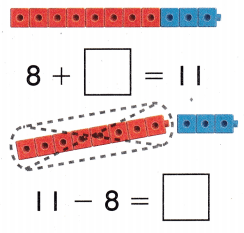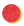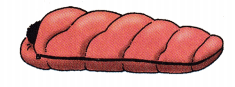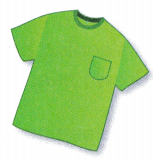# Texas Go Math Grade 1 Lesson 13.4 Answer Key Unknown Numbers

Refer to our Texas Go Math Grade 1 Answer Key Pdf to score good marks in the exams. Test yourself by practicing the problems from Texas Go Math Grade 1 Lesson 13.4 Answer Key Unknown Numbers.

## Texas Go Math Grade 1 Lesson 13.4 Answer Key Unknown Numbers

Explore

Listen to the problem. Useto show the story. Draw to show your work.

FOR THE TEACHER • Read the problem. Calvin has 7 toy cars that are red. He has some blue toy cars. He has 10 toy cars. How many blue toy cars does Calvin have?
Calvin has red toy cars = 7
Total number of toy cars are 10
He has some blue cars.
Total number of blue cars = 10 – 7 = 3

Math Talk
Mathematical Processes

How many toy cars are blue? Explain how you got your answer.

Model and Draw

What are the unknown numbers?Use what you know about related facts to find the unknown parts.
The unknown parts is 3.

Share and Show

Useto find the unknown numbers. Write the numbers.

Question 1.
8 + __________ = 15
15 – 8 = __________
8 + 7 = 15
15 – 8 = 7

Question 2.
13 = 9 + __________
__________ = 13 – 9
13 = 9 + 4
4 = 13 – 9

Question 3.
5 + __________ = 14
14 – 5 = __________
5 + 9 = 14
14 – 5 = 9

Question 4.
14 = 6 + __________
__________ = 14 – 6
14 = 6 + 8
8 = 14 – 6

Question 5.
9 + __________ = 16
16 – 9 = __________
9 + 7 = 16
16 – 9 = 7

Question 6.
17 = 8 + __________
__________ = 17 – 8
17 = 8 + 9
9 = 17 – 8

Problem Solving

Write the unknown numbers. Useif you need to.

Question 7.
7 + __________ = 15
15 – 7 = __________
7 + 8 = 15
15 – 7 = 8

Question 8.
5 + __________ = 11
11 – 5 = __________
5 + 6 = 11
11 – 5 = 6

Question 9.
__________ + 10 = 20
20 – 10 = __________
10 + 10 = 20
20 – 10 = 10

Question 10
__________ + 9 = 16
16 – 9 = __________
7 + 9 = 16
16 – 9 = 7

Question 11.
H.O.T. Solve. Rick has 10 party hats. He needs l hats for his party. How many more party hats does Rick need?_________ party hats

Question 12.
H.O.T. Multi-Step Solve. Useif you need to.
There were 15 children at the park. Some children went home. Then 4 more children came to the park. Now there are 13 children at the park. How many children went home?
__________ children
Total number of children at the park = 15
More children came to park = 4
Total number of children came to park = 19
Now children at the park = 13
Children went to home = 19 – 13 = 6

Use. Choose the correct answer.

Question 13.
Analyze 14 cars are at the starting line. 10 cars cross the finish line. How many cars did not cross the finish line?10 + ________ = 14
(A) 4
(B) 9
(C) 5
Cars at the starting line = 14.
Cars cross the finish line = 10
The cars did not cross the line are 14 – 10 = 4
Option A is the correct answer.

Question 14.
Nick has 3 blue cubes in his hand. The cubes on his desk are yellow. He has 12 cubes. How many yellow cubes does Nick have?3 + _________ = 12
12 – __________ = 3
(A) 6
(B) 9
(C) 4
Total number of cubes near Nick = 12
Nick has blue cubes in his hand = 3
The remaining cubes are yellow colour
Total number of yellow colour cubes = 12 – 3 = 9.
Option B is the correct answer.

Question 15.
Multi-Step Tori counts the toys in her toy box. She has 3 bears and 3 cars. The rest are dolls. She has 12 toys in her toy box. How many dolls does Tori have?(A) 9
(B) 5
(C) 6
Tori has 3 bears and 3 cars.
Total number of toys in the toy box are 12
The rest of the toys are 12 – 3 + 3 = 6.
Option C is the correct answer.

Question 16.
Texas Test Prep What is the unknown number?
9 + _________ = 18
(A) 8
(B) 9
(C) 11
9 is the unknown number.

TAKE HOME ACTIVITY • Hove your child exploin how using subtraction can help him or her find the unknown number in 7 + _________ = 16.
7 + 9 = 16

### Texas Go Math Grade 1 Lesson 13.4 Homework and Practice Answer Key

Write the unknown numbers. Drawif you need to.

Question 1.
9 + _________ = 18
18 – 9 = _________
9 + 9 = 18
18 – 9 = 9

Question 2.
7 + _________ = 15
15 – 7 = _________
7 + 8 = 15
15 – 7 = 8

Question 3.
12 = 8 + _________
_________ = 12 – 8
12 = 8 + 7
7 = 12 – 7

Question 4.
_________ + 6 = 15
15 – 6 = _________
9 + 6 = 15
15 – 6 = 9

Problem Solving

Question 5.
Mr. Brown has 7 sleeping bags. He has 16 campers. How many more sleeping bags does Mr. Brown need?___________ sleeping bags
Mr. Brown has sleeping bags = 7
He has campers = 16
More sleeping bags does Mr. Brown need = 16 – 7 = 9

Lesson Check

Question 6.
14 children are walking to raise money. 8 children finish the walk. How many children did not finish the walk?
8 + ________ = 14
(A) 4
(B) 7
(C) 6
Children are walking to raise money = 14
Children finish the walk = 8
Children did not finish the walk = 14 – 8 = 6

Question 7.
Jasmine has 6 red pencils in her pencil case. The pencils in her hand are blue. She has 13 pencils. How many blue pencils does Jasmine have?
6 + __________ = 13
13 – _________ = 6
(A) 6
(B) 1
(C) 7
Total number of pencils = 13
Jasmine has red pencils in her pencil case = 6
Pencils in her hand are blue.
Number of blue pencils = 13 – 6 = 7
Number of blue pencils are 7.

Question 8.
What is the unknown number?
________ + 8 = 16
(A) 8
(B) 9
(C) 7
8 is the unknown number.

Question 9.
Multi-step Sam has 17 shirts in his drawer. He has 5 red shirts and 4 green shirts. The rest of his shirts are blue. How many blue shirts does Sam have?(A) 9
(B) 8
(C) 10